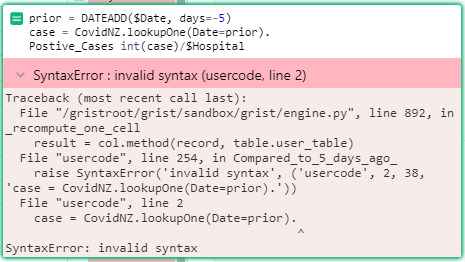# Formula for Offset sort of formula

I’m needing to create a formula to basically compare values. Right now, I want to compare todays hospitalisations to 5 days ago’s cases.

I.e. in Excel, it would be G6 cell would be =D6/C1. Then G7 would be D7/C2.

This document is where I’ve been trying and faililngHi there!

I created a quick example using my Parish’s data. You can view it at the link below:

https://public.getgrist.com/gvUWqfmAHB9Z/Community-637/p/2

Two things to note - make sure your Date column is the Date column type and the numbers columns are all numeric. Otherwise, you may get an error on the formula column.

In the document, I have the following formula in the comparison column.

``````prior = DATEADD(\$Lab_Collection_Date, days=-5)
cases = Covid_Cases.lookupOne(Lab_Collection_Date=prior).Daily_Positive_Test_Count
int(cases)/\$Hospitalizations
``````

First, we need to find the date that was 5 days ago. So, we take the date in the Lab Collection Date column then subtract 5 days.

Next, we need to find the number of positive cases on that day. Format here is `TABLE_NAME.lookupOne(COLUMN_A=prior).COLUMN_B` where Column A is the collection date column and column B is your case count column.

Last, we compare the two! I have int() added so you can format this formula column as a numeric column. this allows you to format your result as a percentage and/or adjust the number of decimals you wish to show using the column configuration panel on the right hand side of your table.

Let me know if you have any questions!

It makes a heap of sense, but seems to still be failing.
Here’s the link too (as not privatehttps://learn.getgrist.com/sSeJ7FRWGWBp/Untitled-document``````prior = DATEADD(\$Date, days=-5)
case = CovidNZ.lookupOne(Date=prior).Positive_Cases
int(case)/\$Hospital
``````

Copy/paste this one.

Positive was spelled incorrectly on .Positive_Cases and was also on the last line but should work now!

Oh my goodness…!!! Thank you! I had gone back and forwards for ages trying to figure out what I’d done wrong!

1 Like